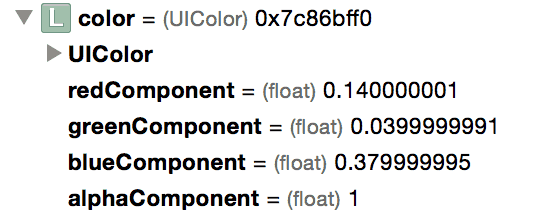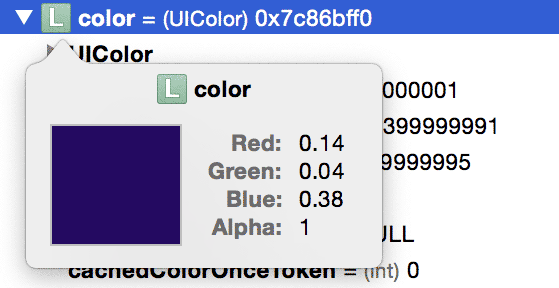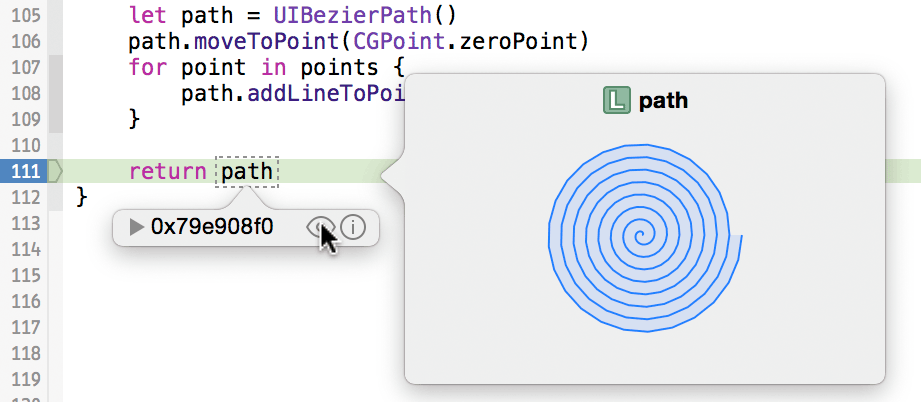# Quick Look DebuggingNate Cook April Peng 🚩🌱Hold 住！并没有试图找出如何将这些组件加在一起的办法。其实有一个更好的办法。``````func buildPathWithRadius(radius: CGFloat, steps: CGFloat, loopCount: CGFloat) -> UIBezierPath {
let away = radius / steps
let around = loopCount / steps * 2 * CGFloat(M_PI)

let points = map(stride(from: 1, through: steps, by: 1)) { step -> CGPoint in
let x = cos(step * around) * step * away
let y = sin(step * around) * step * away

return CGPoint(x: x, y: y)
}

let path = UIBezierPath()
path.moveToPoint(CGPoint.zeroPoint)
for point in points {
}

return path
}
``````
``````- (UIBezierPath *)buildPathWithRadius:(CGFloat)radius steps:(CGFloat)steps loopCount:(CGFloat)loopCount {
CGFloat x, y;
CGFloat away = radius / steps;
CGFloat around = loopCount / steps * 2 * M_PI;

UIBezierPath *path = [UIBezierPath bezierPath];
[path moveToPoint:CGPointZero];

for (int i = 1; i <= steps; i++) {
x = cos(i * around) * i * away;
y = sin(i * around) * i * away;

}

return path;
}
``````### 内置类型

• 图片： `UIImage``NSImage``UIImageView``NSImageView``CIImage`，和 `NSBitmapImageRep` 都可以快速查看。
• 颜色： `UIColor``NSColor`。 （抱歉，`CGColor`。）
• 字符串： `NSString``NSAttributedString`
• 几何： `UIBezierPath``NSBezierPath`，以及 `CGPoint``CGRect`，和 `CGSize`
• 地区 `CLLocation` 将显示一个很大的，互动的映射位置，并显示高度和精度的细节。
• URLs： `NSURL` 将显示 URL 所指的本地或远程的内容。
• 光标： `NSCursor`，为我们中间的光标指示。
• SpriteKit： `SKSpriteNode``SKShapeNode``SKTexture`，和 `SKTextureAtlas` 都会被显示。
• 数据： `NSData` 将漂亮的显示出偏移的十六进制和 ASCII 值。
• 视图： 最后但并非最不重要的，任何 `UIView` 子类都将在快速查看弹出框中显示其内容，方便极了。

### 自定义类型

``````func debugQuickLookObject() -> AnyObject {
``````- (id)debugQuickLookObject {